# Towards Autonomous Driving at the Limit of FrictionDate:18 Jan, 2016

### Towards Autonomous Driving at the Limit of Friction

Controller Description
The vehicle controller consists of four sections: speed pro ler, lateral controller, longitudinal
controller, and a traction control, shown in figure below.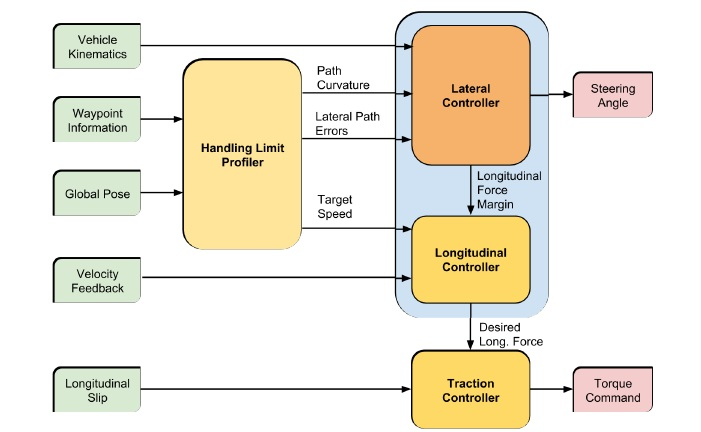The speed pro ler calculates desired velocities based on the path characteristics a certain distance ahead.
The lateral controller uses a full state feedback scheme to reduce the vehicle’s heading error and lateral
error. The concept of Centre of Percussion (COP) is used as reference point to introduce
some stability when tires are slipping. The longitudinal controller monitors the lateral
force required, and uses the spare forces to maximize longitudinal performance. On most
vehicles, a traction controller is a standard equipment; it monitors diff erences in the wheels’
spins to predict slip, and distribute longitudinal power to counter/minimize the slippage.
Many methods can be used to generate a speed pro le for the vehicle. For racing
purposes, the desires paths are generated oine to optimize vehicle performance. Similar
characteristics can be achieved with a sophisticated path planning algorithm. For realtime
path planners, a spline method can be used to calculate the desired velocity. The
paths ahead of the vehicle is tted through a high order piece wise spline; the curvatures
of each piece is compared; and a velocity pro le can be generated for each splines using
equation below.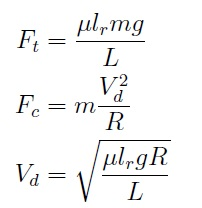For the purpose of controller design, the simplest case is used for veri cations
only. A constant curvature is t of three points ahead of the vehicle. Using the vehicle
coordinates, the lateral distances between the path and three speci ed points ahead (0m,
5m, and 30m)of the vehicle are found. A circle is t through the three points; depending
on the lateral errors, the radius of the circle changes. This radius is used to calculate a
reference velocity based on the centrifugal force and the maximum frictional force supported
by the tires where Ft is the maximum available tire force,  is the coecient of friction, Fc is the
centrifugal force generated around a circle with radius R at the desired velocity Vd.

The lateral controller consists of a feedforward controller and a feedback controller.
The feedforward controller uses the centre of percussion of the rear tires, shown below to simplify calculations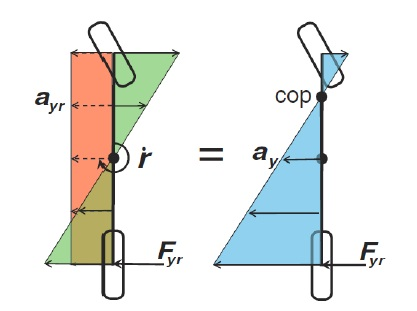The centre of percussion with respect to the reartires is a special point
where the sum of lateral acceleration from the rear tire, ay, is zero.
The rear tires produce two e ects on the vehicle system. They create a lateral acceleration
on the CG, and they produce an angular acceleration about CG. At the COP, these two
e ects cancel out, and the ay from the rear tires is zero.
The feedforward controller takes in current path curvature, path distance and velocity,
and calculate the forces needed to go through a curve.The feedforward
force can be derived below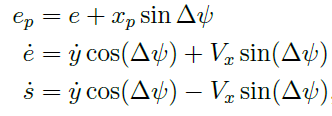where ep is the lateral error a xed distance, xp, away from the CG.  is the heading
error. Vx is the vehicle longitudinal velocity, and s is the distance travelled by the vehicle.
Assuming that Vx  0, using a small angle assumption, the following can be obtained: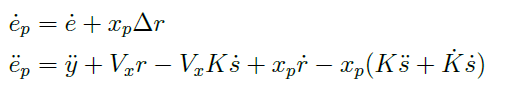where y is the lateral acceleration of the vehicle in vehicle coordinate frame. r is the
vehicle yaw rate, K is the instantaneous curvature of the path, and _K is the change in
curvature.

Substitute in the Single Track Vehicle Model the following
error dynamic can be written: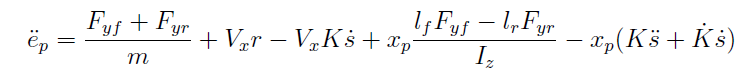Using the concept of centre of percussion, the error dynamics can
be simpli ed.At the COP, the e ect of rear tire forces cancel each other out:Thus, at the centre of percussion, the error dynamics becomes:The feedforward command is intended to reduce the error dynamics to zero; therefore, the
feedforward law is obtained by equating ecop in Eq. (5.7) to zero. The lateral feedforward
force, F_fw is de ned as: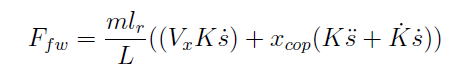The fi rst four feedback states are purely for lane keeping purposes. When regulated
to zero, the vehicle will be tracking the path in the correct heading. The last two terms
act as a future feedback term. These terms aim to start steering early when a curve is
coming up. Much like the human driving behaviour, when a curve is coming up, one would
steer slightly in the direction of the curve, thus reducing the amount of steering needed
otherwise. The two future terms act like a damper on the original feedback system.
The overall feedback law is described below: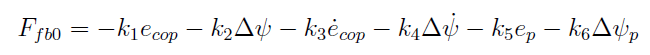It should be noted that in this setup, the feedforward command takes priority, feedback
force ensures that the vehicle is always operating near the limit of friction. With the limit
set, the actual feedback amount is described below:The last task of the lateral controller is that it needs to inform the longitudinal controller
how much tire friction is left, Fm, a similar calculation to the  F is used: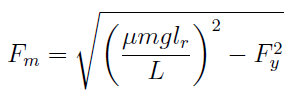The longitudinal controller is a small feedback controller that calculates the desired
longitudinal force, based on the lateral force required and the desired speed pro le.
From the speed pro ler described by Equation (5.1), a desired speed, Vd is obtained.
This speed is fed back via a proportional controller with linear gain kspd: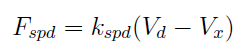where Fspd is the force generated from the desired speed. This force is then compared to
the Fm value calculated in Eq. (5.15), the minimum of the two is selected as the longitudinal
output.When the gains are tuned correctly, simulation has shown that this simple structure
is able to maintain the vehicle near its tire frictions on most paths. Fm ensures that the
vehicle is always going as fast as it can; however, in situations where a sharp curve appears
right after a straight long path, the vehicle would not slow down until it reaches the end of
the path. Fspd ensures that the vehicle slows down to an acceptable speed that can travel
through the turn, before reaching the turn itself.
The last piece in the longitudinal control aspect is a traction controller. The traction
controller monitors the longitudinal slip ratio, and ensures that the desired torque at the
wheel is reached through a proportional controller.

Furthermore, it ensures that the longitudinal slip ratio does not exceed a dangerous
level, in this case, 0.7. The controller would ramp down the acceleration needed if this
threshold is exceeded.

The full thesis may be found here